A Source Book in Mathematics, 1200-1800

Contents:
Author: Isaac Newton  | Date: 1939

Show Summary
Mathematics

4 NEWTON AND GREGORY.

# Binomial Series

### LETTER OF JUNE 13, 1676

Most worthy Sir,

Though the modesty of Mr. Leibniz, in the extracts from his letter which you have lately sent me, pays great tribute to our countrymen for a certain theory of infinite series, about which there now begins to be some talk, yet I have no doubt that he has discovered not only a method for reducing any quantities whatever to such series, as he asserts, but also various shortened forms, perhaps like our own, if not even better. Since, however, he very much wants to know what has been discovered in this subject by the English, and since I myself fell upon this theory some years ago, I have sent you some of those things which occurred to me in order to satisfy his wishes, at any rate in part.

Fractions are reduced to infinite series by division; and radical quantities by extraction of the roots, by carrying out those operations in the symbols just as they are commonly carried out in decinaal numbers. These are the foundations of these reductions: but extractions of roots are much shortened by this theorem,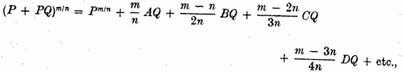where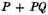signifies the quantity whose root or even any power, or the root of a power, is to be found; P signifies the first term of that quantity, Q the remaining terms divided by the first, and m/n the numerical index of the power of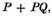whether that power is integral or (so to speak) fractional, whether positive or negative. For as analysts, instead of aa, aaa, etc., are accustomed to write a2, a3, etc., so instead of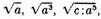etc. I write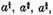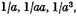I write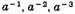.1 And so for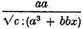I write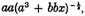and for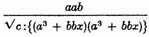I write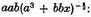in which last case, if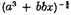is supposed to be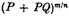in the Rule, then P will be equal to a3, Q to bbx/a3, m to –2, and n to 3. Finally, for the terms found in the quotient in the course of the working I employ A, B, C, D, etc., namely, A for the first term, Pm/n; B for the second term, (m/n)AQ; and so on. For the rest, the use of the rule will appear from the examples.

Example 1.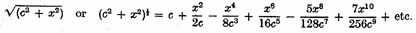For in this ease,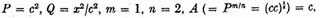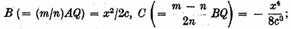and so on.

### LETTER OF OCTOBER 24, 1676

Cambridge October 24 1676

Most worthy Sir,

I can hardly ten with what pleasure I have read the letters of those very distinguished men Leibniz and Tschirnhaus.4 Leibniz’s method for obtaining convergent series is certainly very elegant, and it would have sufficiently revealed the genius of its author, even if he had written nothing else. But what he has scattered elsewhere throughout his letter is most worthy of his reputation—it leads us also to hope for very great things from him. The variety of ways by which the same goal is approached has given me the greater pleasure, because three methods of arriving at series of that kind had already become known to me, so that I could scarcely expect a new one to be communicated to us. One of mine I have described before; I now add another, namely, that by which I first chanced on these series—for I chanced on them before I knew the divisions and extractions of roots which I now use. And an explanation of this will serve to lay bare, what Leibniz desires from me, the basis of the theorem set forth near the beginning of the former letter.

At the beginning of my mathematical studies, when I had met with the works of our celebrated Wallis, on considering the series by the intercalation of which he himself exhibits the area of the circle and the hyperbola, the fact that, in the series of curves whose common base or axis is x and the ordinates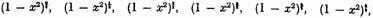etc.,

if the areas of every other of them, namely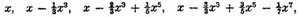etc.

could be interpolated, we should have the areas of the intermediate ones, of which the first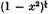is the circle: in order to interpolate these series I noted that in all of them the first term was x and that the second terms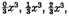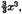etc., were in arithmetical progression, and hence that the first two terms of the series to be intercalated ought to be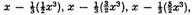etc. To intercalate the rest I began to reflect that the denominators 1, 3, 5, 7, etc. were in arithmetical progression, so that the numerical coefficients of the numerators only were still in need of investigation. But in the alternately given areas these were the figures of powers of the number 11, namely of these, 110, 111, 112, 113, 114, that is, first 1; then 1, 1; thirdly, 1, 2, 1; fourthly 1, 3, 3, 1; fifthly 1, 4, 6, 4, 1, etc. And so I began to inquire how the remaining figures in these series could be derived from the first two given figures, and I found that on putting m for the second figure, the rest would be produced by continual multiplication of the terms of this series,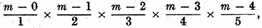etc.

For example, let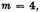and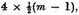that is 6 will be the third term, and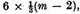that is 4 the fourth, and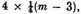that is 1 the fifth, and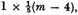that is 0 the sixth, at which term in this case the series stops. Accordingly, I applied this rule for interposing series among series, and since, for the circle, the second term was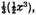I put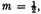and the terms arising were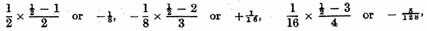and so to infinity. Whence I came to understand that the area of the circular segment which I wanted was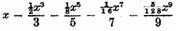etc.

And by the same reasoning the areas of the remaining curves, which were to be inserted, were likewise obtained: as also the area of the hyperbola and of the other alternate curves in this series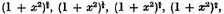etc. And the same theory serves to intercalate other series, and that through intervals of two or more terms when they are absent at the same time. This was my first entry upon these studies, and it had certainly escaped my memory, had I not a few weeks ago east my eye back on some notes.

But when I had learnt this, I immediately began to consider that the terms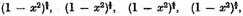etc.,

that is to say,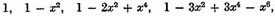etc.

could be interpolated in the same way as the areas generated by them: and that nothing else was required for this purpose hut to omit the denominators 1, 3, 5, 7, etc., which are in the terms expressing the areas; this means that the coefficients of the terms of the quantity to be intercalated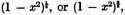or in general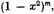arise by the continued multiplication of the terms of this series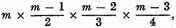etc.,

so that (for example)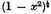was the value of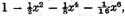etc.,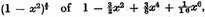etc.,

and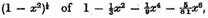etc.

So then the general reduction of radicals into infinite series by that rule, which I laid down at the beginning of my earlier letter, became known to me, and that before I was acquainted with the extraction of roots. But once this was known, that other could not long remain hidden from me. For in order to test these processes, I multiplied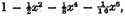etc.

into itself; and it became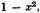the remaining terms vanishing by the continuation of the series to infinity. And even so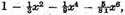etc. multiplied twice into itself also produced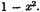And as this was not only sure proof of these conclusions so too it guided me to try whether, conversely, these series, which it thus affirmed to be roots of the quantity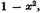might not be extracted out of it in an arithmetical manner. And the matter turned out well. This was the form of the working in square roots.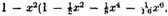etc.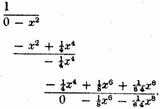### TO FIND THE NUMBER OF A LOGARITHM

Given b, log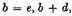log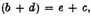it is required to find the number whose logarithm is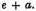Take a series of continual proportions b, d, d2/b, d3/b2, etc., and another series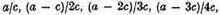etc., and let f/c be the product of the first two terms of the second series, g/c that of the first three, h/c that of the first four, i/c that of the first five, etc. The number whose logarithm is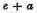will be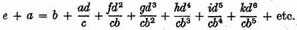Hence with a little work but without difficulty any pure equation whatever may be solved.

1 Newton had learned this method of broken and negative exponents from Wallis, but the idea goes back as far as Oresme and Chuquet; see Selection II.2. Through the influence of Wallis and Newton the method was gradually adopted by other mathematicians. The otation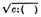indicates the cube root.

2 Kepler, in his Astronomia nova stellae Martis (Heidelberg, 1609), showed that the problem of finding the position of a planet in its elliptical orbit leads to this other problem.

3 Newton’s letters were first published in J. Wallis, Opera mathematica, III (Oxford, 1699), 622–629; the letter of Gregory was first published in Correspondence of scientific men of the X V IIthcentury, ed. S. J. Rigaud (2 vols.; Oxford University Press, Oxford, 1841), II, 209. Gregory, like Newton, used his result to expand many functions into infinite series. He also discovered Taylor’s theorem; see Selection V.11. In a letter to Collins of February 15, 1671, we find as one of his results the series for tan-1x, or, more precisely (since the tangent for Gregory is a line), the expansion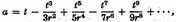if r tan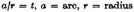(Gregory Memorial Volume, p. 170), first published in Commercium epistoIicum J. Collins et aliorum de analysi promota (London, 1712), 25–26. For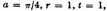we obtain the series of Leibniz. On Collins see p. 290.

4 Tschirnhaus (see Selection II.11) wrote a letter to Oldenburg, dated September 1, 1676, of which Oldenburg informed Newton.

Contents:

### Related Resources

Sir Isaac Newton

Title: A Source Book in Mathematics, 1200-1800

Select an option:

## Email Options

Title: A Source Book in Mathematics, 1200-1800

Select an option: